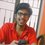# Am I missing something?

I know this question looks simple and might in fact be simple, but, please help me with this one:- If $n$ things are arranged in a circular order, then show that the number of ways of selecting four of the things no two of which are consecutive is $\dfrac{n(n - 5)(n - 6)(n - 7)}{4!}$.

My approach is that since, the things are arranged in circular order, lets select 4 thing such that there is $a,b,c,d$ things between each of them where $a,b,c,d$ are positive integers and satisfy the equation $a + b + c + d = n-4$ which can be obtained by $\dbinom{n-4-1}{4-1} = \dfrac{(n-5)(n-6)(n-7)}{3!}$ but the required answer is $\dfrac{n}{4}$ times this answer. Where am I going wrong, a detailed solution is appreciated.Note by Ashish Menon
4 years, 10 months ago

This discussion board is a place to discuss our Daily Challenges and the math and science related to those challenges. Explanations are more than just a solution — they should explain the steps and thinking strategies that you used to obtain the solution. Comments should further the discussion of math and science.

When posting on Brilliant:

• Use the emojis to react to an explanation, whether you're congratulating a job well done , or just really confused .
• Ask specific questions about the challenge or the steps in somebody's explanation. Well-posed questions can add a lot to the discussion, but posting "I don't understand!" doesn't help anyone.
• Try to contribute something new to the discussion, whether it is an extension, generalization or other idea related to the challenge.
• Stay on topic — we're all here to learn more about math and science, not to hear about your favorite get-rich-quick scheme or current world events.

MarkdownAppears as
*italics* or _italics_ italics
**bold** or __bold__ bold
- bulleted- list
• bulleted
• list
1. numbered2. list
1. numbered
2. list
Note: you must add a full line of space before and after lists for them to show up correctly
paragraph 1paragraph 2

paragraph 1

paragraph 2

[example link](https://brilliant.org)example link
> This is a quote
This is a quote
    # I indented these lines
# 4 spaces, and now they show
# up as a code block.

print "hello world"
# I indented these lines
# 4 spaces, and now they show
# up as a code block.

print "hello world"
MathAppears as
Remember to wrap math in $$ ... $$ or $ ... $ to ensure proper formatting.
2 \times 3 $2 \times 3$
2^{34} $2^{34}$
a_{i-1} $a_{i-1}$
\frac{2}{3} $\frac{2}{3}$
\sqrt{2} $\sqrt{2}$
\sum_{i=1}^3 $\sum_{i=1}^3$
\sin \theta $\sin \theta$
\boxed{123} $\boxed{123}$

## Comments

Sort by:

Top Newest

Hi @Ashish Siva The first answer is the correct one. Without going into too much math here, I think your approach is correct only if you fix one of the objects as a selected one. In reality all $n$ objects can be either selected or not. So, this will be a subset of the complete solution.

For example if you have $9$ objects, there are $9$ ways to select the objects. (consistent with the first answer). However, if you pick one as selected, then there are only $4$ ways to pick the objects (consistent with your answer).

Make sense?

- 4 years, 10 months ago

Log in to reply

Ya, thanks! Surely makes sense!

- 4 years, 10 months ago

Log in to reply

@Geoff Pilling @Hung Woei Neoh @Sambhrant Sachan please do comment.

- 4 years, 10 months ago

Log in to reply

×

Problem Loading...

Note Loading...

Set Loading...